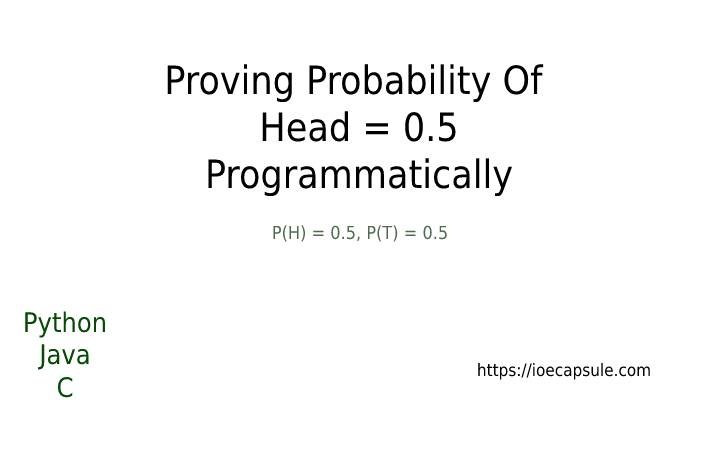### Proving Coin Toss Probability ProgrammaticallyC

If you toss a coin, you will get either head or a tail. Meaning that there is 50-50 chance of getting head or tail. Theoretically, the probability of getting head/tail is thus 0.5.

But, it is not the case that you will get 50% of head and 50% of tail in the same single toss, it will be either head or tail. This makes the probability exactly either 1 or 0 for a single toss. The question here is how do you obtain 0.5 probability if it keeps giving the same result for some tosses.

Consider a scenario. You are tossing a coin 5 times separately. Suppose you got heads in all 5 tosses. This means that the probability of getting head is 1 because you get head in every toss. What if you got all tails ? The probability of head would be 0 in this case. Another case would be you got 2 heads out of 5 tosses which would result in probability of head of 2/5 i.e.0.4. Still, why are we not getting 0.5 as probability of head ? The answer is given below.

The probability we are saying 0.5 is a theoretical value. This value can not be exact in real scenarios. Rather, it is obtained by performing a lot of sample experiments and calculating the probability. If you perform single, double or even 5-10 tosses, it is not guaranteed that you will obtain 0.5 probability of heads or tails. You will have to perform as many as 100s or even 1000s tosses and then the value will converge to 0.5. To prove this statement, let’s write some coin toss program in some languages.

## How to prove that probability of head is 0.5 programmatically ?

1. Set number of tosses to a variable N
2. Loop through N times, generate random integer between [0, 1] inclusive and increment headCount if it is 1
3. Divide total number of heads by total number of tosses N
4. The idea is that if you take large enough N, the probability will converge to the value of 0.5

## How to prove that probability of head is 0.5 in python ?

``````import random;

for i in range(0, N):
if(random.randrange(0, 2) == 1):

N = 1
while N > 0:
N = int(input('Enter number of flips: \n'))
try:
print('P(H) = ', probalility, end='\n')
except ZeroDivisionError:
print('exiting...')
``````

## How to prove that probability of head is 0.5 in Java ?

``````import java.util.Random;
import java.util.Scanner;
class Main{
public static void main(String[] args){
Scanner scanner = new Scanner(System.in);
int n;
do{
System.out.println("Enter number of tosses: ");
n = scanner.nextInt();
try{
System.out.println("P(H) = " + probability);
}catch(Exception ex){
System.out.println("Exiting..");
}
}while(n > 0);
}

Random random = new Random();
for(int i = 0; i < n; i++){
if((Math.abs(random.nextInt() % 10)) >4){
}
}
}
}``````

## How to prove that probability of head is 0.5 in c ?

``````#include <stdio.h>

for(int i = 0; i < n; i++){
if((rand() % 10) > 4){
}
}
}

int main()
{
int n;
do{
printf("Enter number of flips: \n");
scanf("%d", &n);
if(n <= 0){
printf("Exiting..");
break;
}
printf("P(H) = %f\n", probability);
}while(n > 0);

return 0;
}
``````

So, these were some example programs. We have used basically two methods for determining head counts. The first one is generating random integer value between [0, 1] inclusive and considering 1 as head, 0 as tail. Another approach is generating random integer between 0 to 9 inclusive and considering lower numbers less or equal than 4 as tail and upper numbers greater than 4 as head. You can follow whatever approach you find suitable. The roulette wheel approach is also well known approach for the cases like this one.

HAPPY CODING !